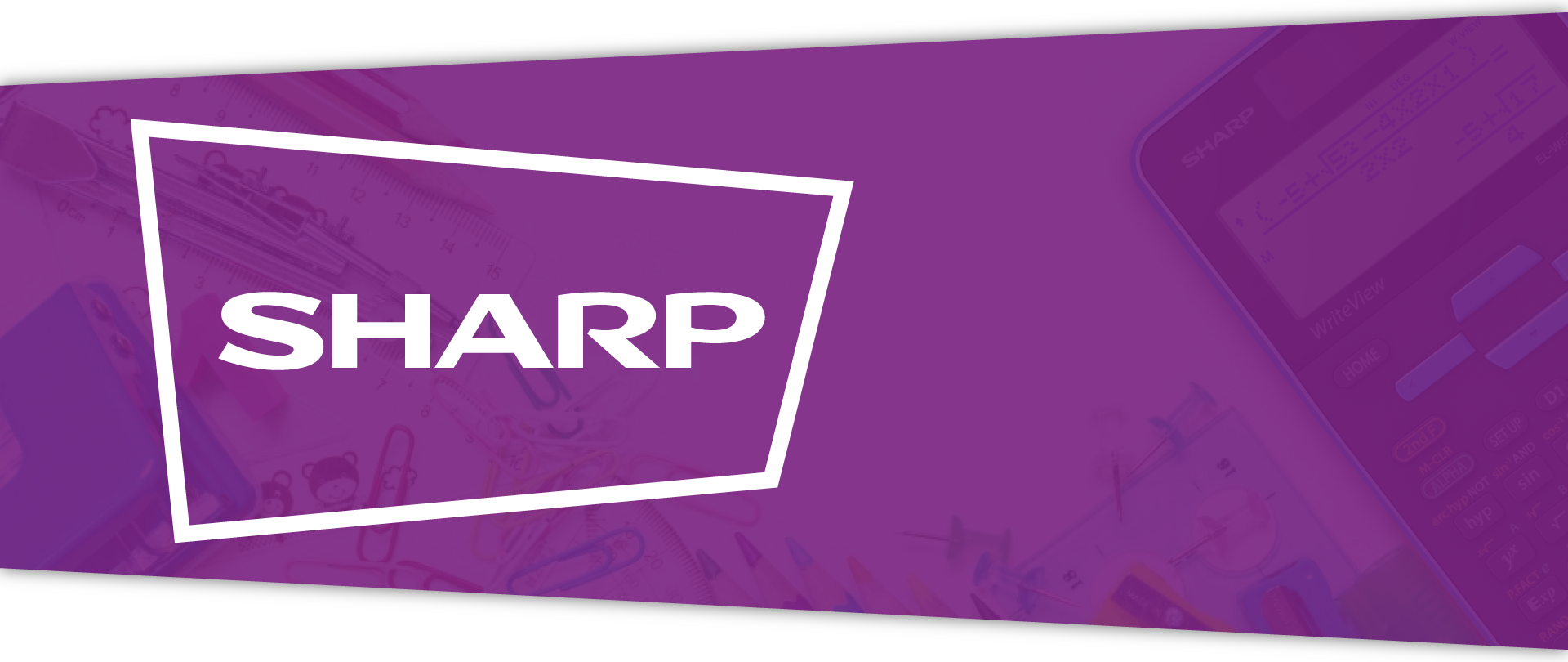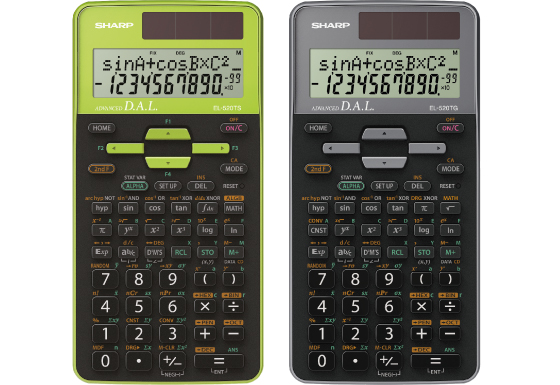## EL-520TG/TS

The EL-520TG in gray color offers over 400 built-in functions and the EL-520TS in green color performs 420 statistical, trigonometric and mathematical functions. Both models have a large 2-line 10+2 digits display, dual power and come with a hard cover.## Specification EL-520TG

• Built-In Functions: 400+
• Entry System: Algebraic (D.A.L.)
• Power Source: Solar & Battery
• Dimensions and Weight: 158 × 80 × 14 mm, 91 g

Mathematical Functions

• Fractions
• Numeric Derivative and Integral
• Complex Number Calculation

Memory

• Multi-Line Playback
• Memory Variables: 9

Notation and Decimal Places

• FSE Display (SCI, FIX, ENG)
• 3-Digit Punctuation

Statistics

• 1-Variable Statistics
• 2-Variable Statistics
• Regression Calculations: 6
• Mean, Standard Deviation
• nCr, nPr, n!
• Random Numbers

Trigonometric Functions

• Sin, Cos, Tan and Inverses
• Hyperbolic Functions
• Sexagesimal and Decimal Conversions
• Rectangular and Polar Coordinates Conversions

Number Systems

• Number Systems: DEC, BIN, OCT, HEX, PEN
• Logical Operation (AND, OR, NOT, NEG, XOR)

Scientific Functions

• Metric Conversions
• Physical Constants

## Specification EL-520TS

• Built-In Functions: 420
• Entry System: Algebraic (D.A.L.)
• Power Source: Solar & Battery
• Dimensions and Weight: 161 × 80 × 15 mm, 110 g

Mathematical Functions

• Fractions
• Numeric Derivative and Integral
• Complex Number Calculation

Memory

• Multi-Line Playback
• Memory Variables: 9

Notation and Decimal Places

• FSE Display (SCI, FIX, ENG)
• 3-Digit Punctuation

Statistics

• 1-Variable Statistics
• 2-Variable Statistics
• Regression Calculations: 6
• Mean, Standard Deviation
• nCr, nPr, n!
• Random Numbers

Trigonometric Functions

• Sin, Cos, Tan and Inverses
• Hyperbolic Functions
• Sexagesimal and Decimal Conversions
• Rectangular and Polar Coordinates Conversions

Number Systems

• Number Systems: DEC, BIN, OCT, HEX, PEN
• Logical Operation (AND, OR, NOT, NEG, XOR)

Scientific Functions

• Metric Conversions
• Physical Constants